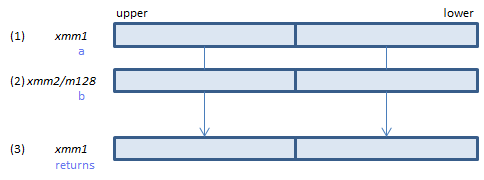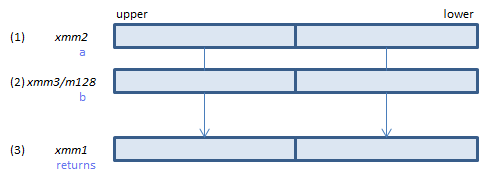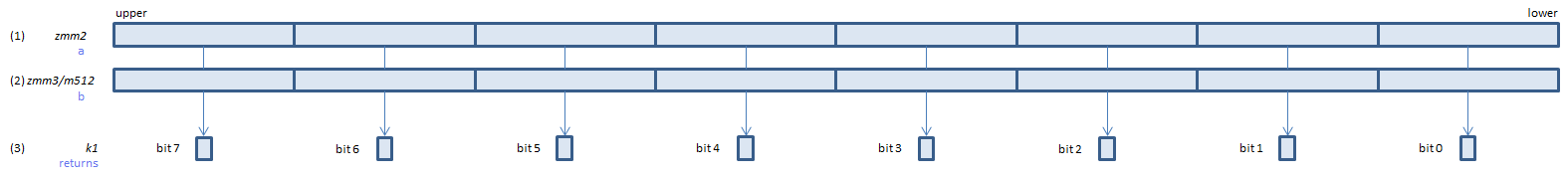﻿ pcmpeqq

## PCMPEQQ - Packed CoMPare EQual Qword

PCMPEQQ xmm1, xmm2/m128    (S4.1
__m128i _mm_cmpeq_epi64(__m128i a, __m128i b)For each QWORD, if (1) == (2) set 1, else set 0, to all bits in the corresponding QWORD of (3).
VPCMPEQQ xmm1, xmm2, xmm3/m128    (V1
__m128i _mm_cmpeq_epi64(__m128i a, __m128i b)For each QWORD, if (1) == (2) set 1, else set 0, to all bits in the corresponding QWORD of (3).
VPCMPEQQ ymm1, ymm2, ymm3/m256    (V2
__m256i _mm256_cmpeq_epi64(__m256i a, __m256i b)For each QWORD, if (1) == (2) set 1, else set 0, to all bits in the corresponding QWORD of (3).
VPCMPEQQ k1{k2}, xmm2, xmm3/m128/m64bcst    (V5+VLFor each QWORD, if (1) == (2) set 1, else set 0, to the corresponding bit of (3).
If k2 bit is 0, the comparison is not done and the corresponding bit of (3) is set to zero. Upper bits of (3) are zero cleared.
VPCMPEQQ k1{k2}, ymm2, ymm3/m256/m64bcst    (V5+VL